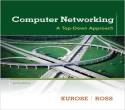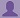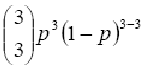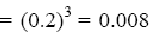SHARE
HELP

To start with, Sr2Jr’s first step is to reduce the expenses related to education. To achieve this goal Sr2Jr organized the textbook’s question and answers. Sr2Jr is community based and need your support to fill the question and answers. The question and answers posted will be available free of cost to all.# Computer Networking : A Top-down Approach

Authors:
James F. Kurose, Keith W. Ross
Exercise:
Review
Chapter:
Computer Networks And The Internet
Edition:
6
ISBN:
9780132856201
Question:
13
 Previous Next

###Question

Suppose users share a 2 Mbps link. Also suppose each user transmits continuously at 1 Mbps when transmitting, but each user transmits only 20 percent of the time. (See the discussion of statistical multiplexing in Section 1.3.)

a. When circuit switching is used, how many users can be supported?

b. For the remainder of this problem, suppose packet switching is used. Why will there be essentially no queuing delay before the link if two or fewer users transmit at the same time? Why will there be a queuing delay if three users transmit at the same time?

c. Find the probability that a given user is transmitting.

d. Suppose now there are three users. Find the probability that at any given time, all three users are transmitting simultaneously. Find the fraction of time during which the queue grows.

###Answer

a) 2 users can be supported because each user requires half of the link bandwidth.

b) Since each user requires 1Mbps when transmitting, if two or fewer users transmit simultaneously, a maximum of 2Mbps will be required. Since the available bandwidth of the shared link is 2Mbps, there will be no queuing delay before the link. Whereas, if three users transmit simultaneously, the bandwidth required will be 3Mbps which is more than the available bandwidth of the shared link. In this case, there will be queuing delay before the link.

c) Probability that a given user is transmitting = 0.2

d) Probability that all three users are transmitting simultaneously =Since the queue grows when all the users are transmitting, the fraction of time during which the queue grows (which is equal to the probability that all three users are transmitting simultaneously) is 0.008.

### Discussions

Post the discussion to improve the above solution.